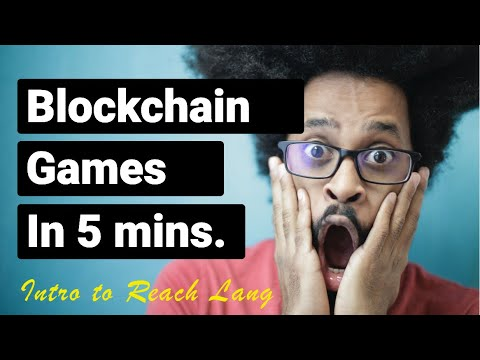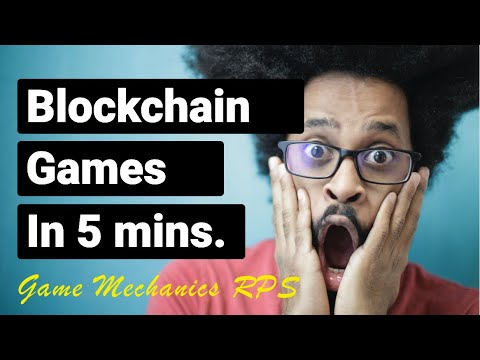TutorialsIntermediate · 15 minutes or less

# Algorand Blockchain Development using Reach Part 1

Software Development is hard. Blockchain development is even harder. With Reach the last statement is false. In this tutorial, I’m going to show Algorand developers how they can start using Reach for their Algorand and future cross-co chain development.

## Requirements

• [Reach SDK] Algorand - Ethereum SDK

## Background

Before starting this tutorial, we will need to install and make sure Reach is configured for Algorand Blockchain Development. For those using Unix based systems make sure the following commands run without error.

`````` \$ make --version
\$ docker --version
\$ docker-compose --version
``````

Tip

If you are using Windows, consult [Reach SDK Installtion Windows]

Once you have confirmed that the above is installed, we can choose a directory for our sample AlgoReach project.

``````mkdir -p ~/reach/AlgoReach && cd ~/reach/AlgoReach
``````

Next, we can install Reach from [GitHub] in our console lets run the following command in our terminal.

`````` curl https://raw.githubusercontent.com/reach-sh/reach-lang/master/reach -o reach ; chmod +x reach
``````

Now, we can check and see if everything worked by running the following command in our terminal.

``````./reach version
``````

Reach is Docker Container, when we first use it, you will need to download the images it uses. You can get these by running the following command in your terminal.

``````./reach update
``````

Congratulations, if you have made it this far without any error run the folowing command in your terminal

``````./reach compile --help
``````

## Steps

### Reach Programming Basics

Reach files have the `.rsh` extension. In you editor of choice lets create our first Reach program called `index.rsh`. Once you have created it let us type the following Reach program.

### Creating our Reach dApp

``````'reach 0.1';
``````

The above code indicates that this is a Reach program

``````export const main = Reach.App(
``````

The above code is the main entry point of our Reach program

``````  {}, [['Alice', {}], ['Bob', {}]],
``````

The above code shows the participants in this program, Alice and Bob.

``````    (A, B) => {
// ...
exit(); });
``````

The above code binds Reach indentifiers A == Alice and B == Bob and defines the body of our program

That is all we need to have a Smart Contract that binds two participants together. The greatest thing about programming in Reach is that the programmer doesn’t need to worry about all the low level workings of a consensus network. All the reach programmer needs to worry about is WHAT participants CANNOT do on a consensus network. This is really important to remember in Blockchain development.

### Creating a Smart Contract Test Runner

Next, we will create a Test Runner for our Reach programs. Let us create a Javascript frontend. We will create a new file named `index.mjs` and add the following.

``````import {loadStdlib} from '@reach-sh/stdlib';
import * as backend from './build/index.main.mjs';
``````

The above code - 1 imports the Reach standard library loader
The above code -2 imports your backend, which `./reach compile` will produce.

``````(async () => {
``````

The above code defines an asynchronous function that will be the body of our frontend.

``````  const stdlib = await loadStdlib();
``````

The above code loads the standard library dynamically based on the `REACH_CONNECTOR_MODE` environment variable.

``````  const startingBalance = stdlib.parseCurrency(100);
``````

The above code defines a quantity of network tokens as the starting balance for each test account.

``````  const alice = await stdlib.newTestAccount(startingBalance);
const bob = await stdlib.newTestAccount(startingBalance);
``````

The above two lines of code create test accounts with initial endowments for Alice and Bob. This will only work on the Reach-provided developer testing network.

``````  const ctcAlice = alice.deploy(backend);
``````

The above code has Alice deploy the application.

``````  const ctcBob = bob.attach(backend, ctcAlice.getInfo());
``````

The above code has Bob attach to it.

``````  await Promise.all([
backend.Alice(stdlib, ctcAlice, {
...stdlib.hasRandom
}),
``````

The above code initialize Alice’s backend.

``````    backend.Bob(stdlib, ctcBob, {
...stdlib.hasRandom
}),
``````

The above code initialize Bob’s backend

``````  ]);

console.log('Hello, Alice and Bob!');
})();
``````

The code above calls this asynchronous function that we defined. Now we are ready to compile our first Reach Algorand dApp.

### Compiling our AlgoReach project

``````./reach compile
``````

### Running our AlgoReach project

Now we are ready to run our First Algo Reach dApp. We will use the following command in our terminal

``````REACH_CONNECTOR_MODE=ALGO REACH_DEBUG=1 ./reach run
``````

You will see much information about the what Algorand blockchain is doing behinds the scenes. If you have made this far, you are ready to start you journey into Cross Chain development with Reach.

Tip

To create this starter project. Use the command below to create a base starter project.

``````./reach init
``````

### Completed AlgoReach project

Full Source
`index.rsh`

``````'reach 0.1';

export const main = Reach.App(
{}, [['Alice', {}], ['Bob', {}]],
(A, B) => {
// ...
exit(); });
``````

`index.mjs`

``````import {loadStdlib} from '@reach-sh/stdlib';
import * as backend from './build/index.main.mjs';

(async () => {
const startingBalance = stdlib.parseCurrency(100);

const alice = await stdlib.newTestAccount(startingBalance);
const bob = await stdlib.newTestAccount(startingBalance);

const ctcAlice = alice.deploy(backend);
const ctcBob = bob.attach(backend, ctcAlice.getInfo());

await Promise.all([
backend.Alice(stdlib, ctcAlice, {
...stdlib.hasRandom
}),
backend.Bob(stdlib, ctcBob, {
...stdlib.hasRandom
}),
]);

console.log('Hello, Alice and Bob!');
})();
``````

Here is a video showing the whole process.Want to take this tutorial further? This example shows in less than 5 minutes how you can implement Rock paper scissors for both Algorand and Ethereum.November 12, 2020